# Haste makes waste

1. 参考网络课程：Python网络爬虫与信息提取
3. 关于正则表达式，在这里也有涉及：正则表达式
4. 正则表达式online check工具 https://regexr.com/
5. 莫烦python-正则表达式

# 0. 快速参考

• 参考1：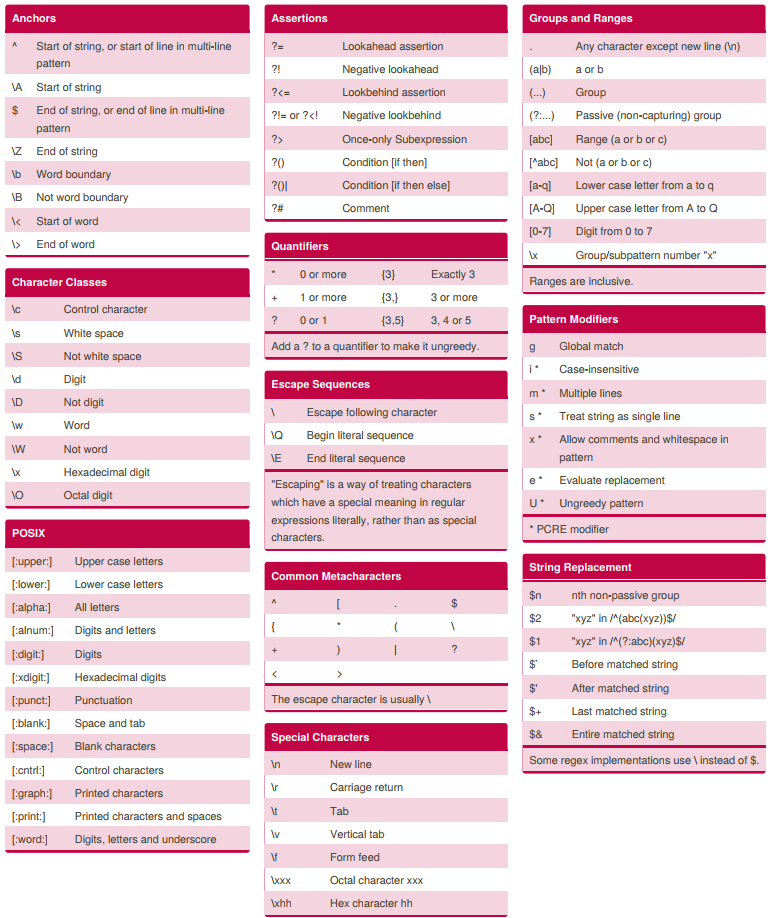• 参考2：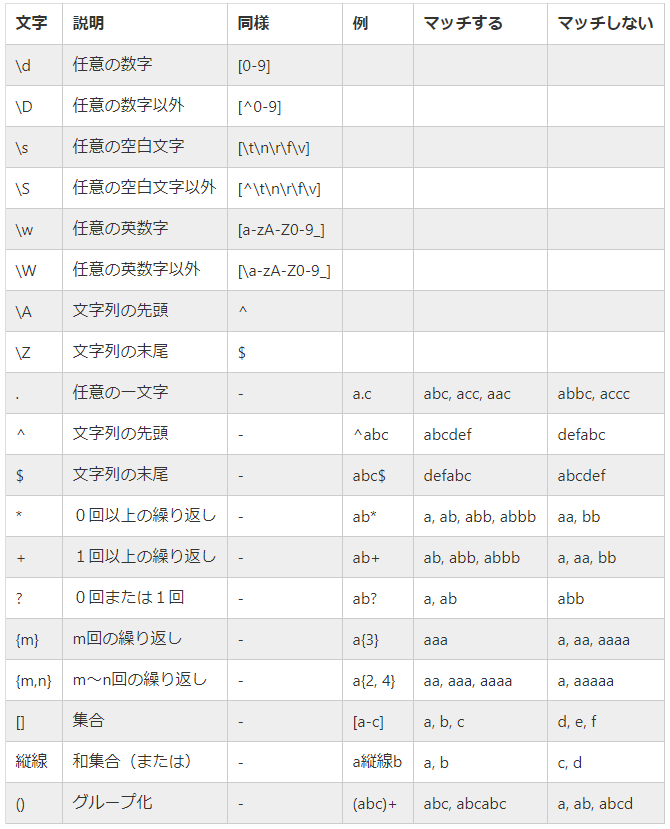# 1. Re(正则表达式)库入门

``````'PN'
'PYN'
'PYTN'
'PYTHN'
'PYTHON'
``````

``````regex='P(Y|YTH|YTHO)?N'
p=re.compile(regex)
``````

## 1.1 正则表达式的常用操作符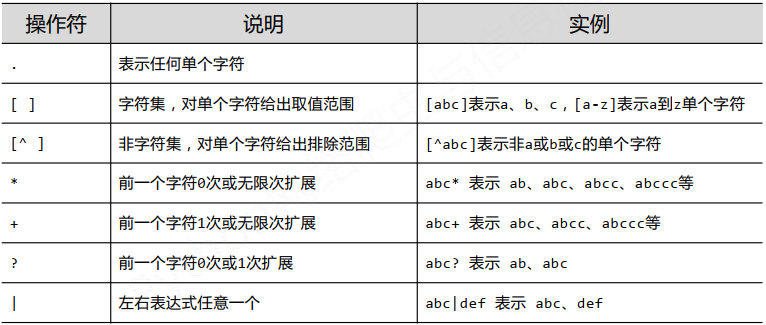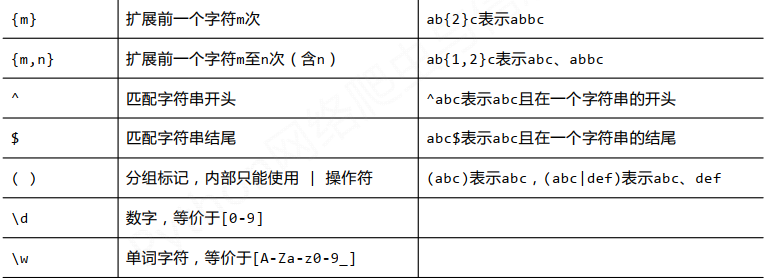• 基本语法示例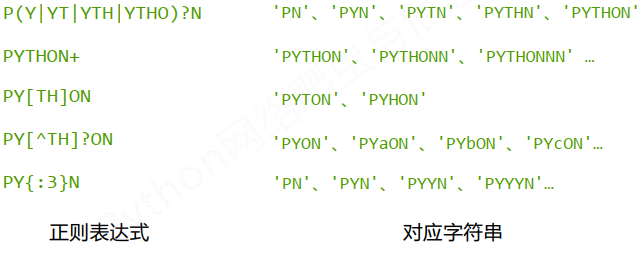• 经典的正则表达式示例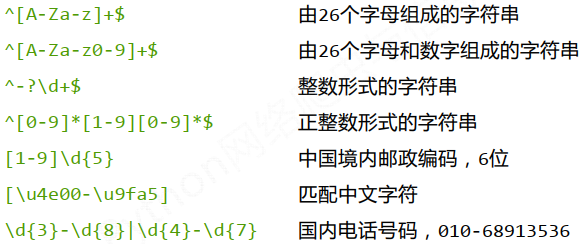# 2. Re库的基本使用

Re库采用raw String原生字符串，书写为`r'text'`，通过这种方式会忽略掉转义符号。比如 `r'[1-9]\d{5}' `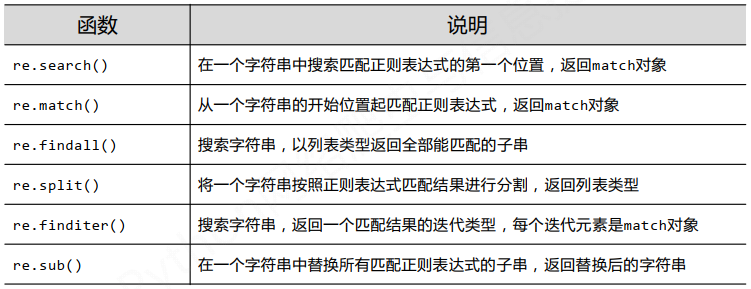## 2.1 search的使用

``````re.search(pattern,string,flags=0)
``````

1. pattern: 正则表达式的字符串或原生字符串
2. string：待匹配字符串
3. flags：正则表达式使用时的控制标记
1. re.I,re.IGNORECASE: 忽略正则表达式的大小写，`[A-Z]`能够匹配小写字符
2. re.M,re.MULTILINE: 正则表达式中的`^`能够将给定字符串的每行当做匹配开始
3. re.S,re.DOTALL：正则表达式中的`.`能匹配所有字符，默认匹配换行外的所有字符
``````import re
match = re.search(r'\d{5}','BIT 10081')
if match:
print(match.group(0))
``````

``````10081
``````

## 2.2 match的使用

``````re.match(pattern,string,flags=0)
``````

1. pattern: 正则表达式的字符串或原生字符串
2. string：待匹配字符串
3. flags：正则表达式使用时的控制标记
1. re.I,re.IGNORECASE: 忽略正则表达式的大小写，`[A-Z]`能够匹配小写字符
2. re.M,re.MULTILINE: 正则表达式中的`^`能够将给定字符串的每行当做匹配开始
3. re.S,re.DOTALL：正则表达式中的`.`能匹配所有字符，默认匹配换行外的所有字符
``````import re
match = re.match(r'\d{5}','10081 BIT ')
if match:
print(match.group(0))
``````

``````10081
``````

## 2.3 findall的使用

``````re.findall(pattern,string,flags=0)
``````

1. pattern: 正则表达式的字符串或原生字符串
2. string：待匹配字符串
3. flags：正则表达式使用时的控制标记
1. re.I,re.IGNORECASE: 忽略正则表达式的大小写，`[A-Z]`能够匹配小写字符
2. re.M,re.MULTILINE: 正则表达式中的`^`能够将给定字符串的每行当做匹配开始
3. re.S,re.DOTALL：正则表达式中的`.`能匹配所有字符，默认匹配换行外的所有字符
``````import re
match = re.findall(r'\d{5}','10081 BIT TSU10084')
print("1:",match)
``````

``````1: ['10081', '10084']
``````

## 2.4 split的使用

``````re.split(pattern,string,maxsplit=0,flags=0)
``````

1. pattern: 正则表达式的字符串或原生字符串
2. string：待匹配字符串
3. maxsplit: 最大分个数，剩余部分作为最后一个元素输出
4. flags：正则表达式使用时的控制标记
1. re.I,re.IGNORECASE: 忽略正则表达式的大小写，`[A-Z]`能够匹配小写字符
2. re.M,re.MULTILINE: 正则表达式中的`^`能够将给定字符串的每行当做匹配开始
3. re.S,re.DOTALL：正则表达式中的`.`能匹配所有字符，默认匹配换行外的所有字符
``````import re
match1 = re.split(r'\d{5}','10081BIT 10084 TSU ')
match2 = re.findall(r'\d{5}','10081 BIT TSU10084')
print("1:",match1)
print("2:",match2)
``````

``````1: ['', 'BIT ', ' TSU ']
2: ['10081', '10084']
``````

## 2.5 finditer的使用

``````re.finditer(pattern,string,flags=0)
``````

1. pattern: 正则表达式的字符串或原生字符串
2. string：待匹配字符串
3. flags：正则表达式使用时的控制标记
1. re.I,re.IGNORECASE: 忽略正则表达式的大小写，`[A-Z]`能够匹配小写字符
2. re.M,re.MULTILINE: 正则表达式中的`^`能够将给定字符串的每行当做匹配开始
3. re.S,re.DOTALL：正则表达式中的`.`能匹配所有字符，默认匹配换行外的所有字符
``````import re
for m in re.finditer(r'\d{5}','BIT10081 TSU10099'):
if m:
print(m.group(0))
``````

``````10081
10099
``````

## 2.6 sub的使用

``````re.sub(pattern,repl,string,count=0,flags=0)
``````

1. pattern: 正则表达式的字符串或原生字符串
2. repl:替换匹配字符串的字符串
3. string：待匹配字符串
4. count：匹配最大替换次数
5. flags：正则表达式使用时的控制标记
1. re.I,re.IGNORECASE: 忽略正则表达式的大小写，`[A-Z]`能够匹配小写字符
2. re.M,re.MULTILINE: 正则表达式中的`^`能够将给定字符串的每行当做匹配开始
3. re.S,re.DOTALL：正则表达式中的`.`能匹配所有字符，默认匹配换行外的所有字符
``````import re
match = re.sub(r'\d{5}',':zipcode','BIT10081 TSU10099')
print(match)
``````

``````BIT:zipcode TSU:zipcode
``````

## 2.7 Re库的另一种等价用法

``````regex = re.compile(pattern,flags=0)
``````

1. pattern: 正则表达式的字符串或原生字符串
2. repl:替换匹配字符串的字符串
• 函数式用法：
``````rst = re.search(r'\d{5}','BIT10081 TSU10099')
``````
• 面向对象用法，编译后的多次操作
``````pat = re.compile(r'\d{5}')
rst = pat.search('BIT10081 TSU10099')
``````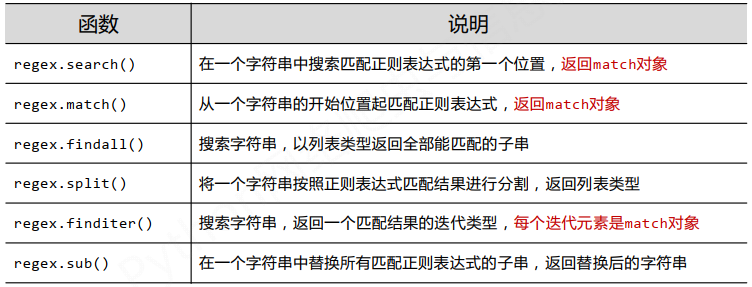# 3. Re库的Match对象

• Match对象的属性
• Match对象的方法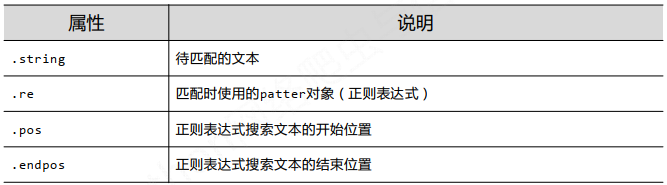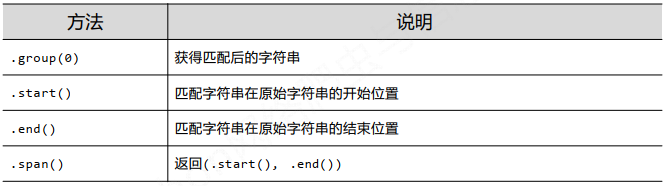``````import re
m = re.search(r'\d{5}','ABCDE10081 F10099')
print("1.string   :  ",m.string)
print("2.pos      :  ",m.pos)
print("3.endpos   :  ",m.endpos)
print("4.group    :  ",m.group(0))
print("5.start    :  ",m.start())
print("6.end      :  ",m.end())
print("7.span     :  ",m.span())
``````

``````1.string   :   ABCDE10081 F10099
2.pos      :   0
3.endpos   :   17
4.group    :   10081
5.start    :   5
6.end      :   10
7.span     :   (5, 10)
``````

# 4. Re库的贪婪匹配和最小匹配

Re库默认采用贪婪匹配，即输出匹配最长的子串。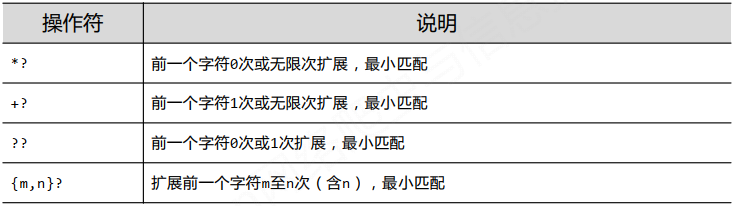``````match1 = re.search(r'PY.*N','PYANBNCNDN')
print("贪婪匹配:",match1.group(0))

match2 = re.search(r'PY.*?N','PYANBNCNDN')
print("最小匹配:",match2.group(0))
``````

``````贪婪匹配: PYANBNCNDN

``````